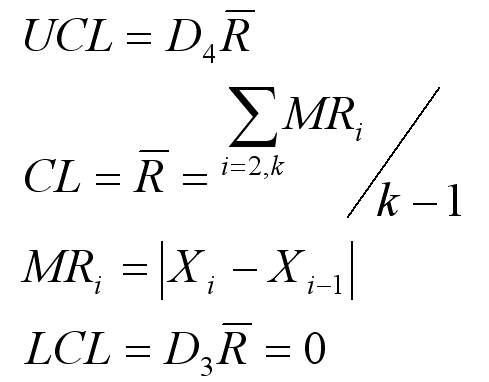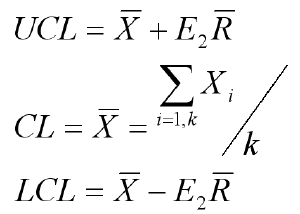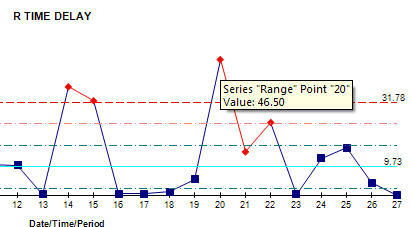# XmR Individuals Chart Formulas

The XmR (Individuals and Moving Range) Chart can help you evaluate a process when there is only one measurement and they are farther apart: monthly postage expense and so on.

Here are the formulas used to calculate both the Moving Range and Individuals chart in QI Macros.

### Moving Range Chart formula### Individuals X Chart formulak = number of data points

for n=2 observations (moving range is calculated from two samples, the present minus the previous value)

D4 = 3.267

D3 = 0

E2 = 2.660

Conforms with ANSI/ASQC B1, B2, B3 1996

### QI Macros XmR Charts employ the Nelson Rule for Moving Range

"Any moving range (Ri) greater than 3.5*Rbar should be removed from the average moving range calculation."

In example below, point 20 (46.50) is greater than 3.5*11.14 (original Rbar), so it will be removed from Rbar Calculation which changes Rbar to 9.73 and UCL/LCL for both the X and R charts.

Source: Control Charts for Individual Measurements, Lloyd S. Nelson, Journal of Quality Technology, Vol. 14, No. 34, July 1982.Process: Calculate, plot, and evaluate the range chart first. If it is "out of control," so is the process. If the range chart looks okay, then calculate, plot, and evaluate the X Chart.

### Other Resources

The XmR Individuals chart is just one of the tools included in the QI Macros SPC Software for Excel.

### Why Choose QI Macros Over Other Control Chart Software?### Affordable

• Only \$299 USD - less with quantity discounts
• No annual subscription fees
• Free technical support### Easy to Use

• Works right in Excel
• Create a chart in seconds
• Easy to customize & share charts### Proven and Trusted

• More than 100,000 users
• In More than 80 countries
• Five Star CNET Rating - Virus free